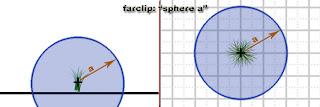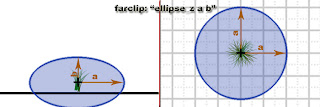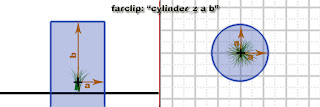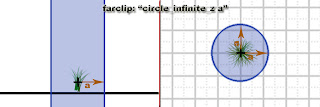## Friday, January 23, 2009

### Using far-clipping part 3

List of all clipping form

In all the following example, "a" and "b" are arbitrary integer.
The first integer "a" always affect the most general setting of the form, while the seconde integer "b" (if any) affect an alternate setting of the form. If the form has x, y and z variations, the second integer "b" (if any) is always a distance along this axis. For example, "box_y 128 256" means 128 along x-axis and z-axis, and 256 along y-axis.

Forms that have X, Y and Z axis variations are only explained for the Z-axis one, but it is easy to figure out how does it work for X-axis and Y-axis variation ;)

The left part of each image is for the side view, the right part is for the top view.

spherecubeellipse_x, ellipse_y, ellipse_zbox_x, box_y, box_zcylinder_x, cylinder_y, cylinder_zcone_x, cone_y, cone_zpyramid_x, pyramid_y, pyramid_zcircle_infinite_x, circle_infinite_y, circle_infinite_zsquare_infinite_x, square_infinite_y, square_infinite_z